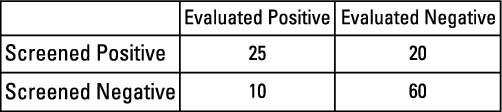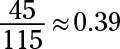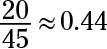##### Statistics: 1001 Practice Problems For Dummies (+ Free Online Practice)The wording of the problems for two-way tables can be extremely tricky; one small change in wording can lead to a totally different answer. Practice as many problems as you can.

## Sample questions

1. Suppose that you have a 2-x-2 table displaying values on gender (male or female) and laptop computer ownership (yes or no) for 100 male and 100 female college students.

Overall, 75% of the students own laptops; 85% of the male students own laptops, as do 65% of the female students. Are gender and laptop ownership independent in this data set?

A. Yes, because the overall ownership rate is 75%.

B. Yes, because the conditional and marginal probabilities are equal.

C. No, because the ownership rates differ by gender.

D. No, because the marginal ownership rate differs from the conditional ownership rates.

E. Choices (C) and (D)

Answer: E. Choices (C) and (D) (No, because the ownership rates differ by gender; no, because the marginal ownership rate differs from the conditional ownership rates.)

If two variables were independent, all three percentages given would have to be equal. The conditional probability of ownership (0.75) would be the same as the marginal probabilities of ownership (0.85 for males; 0.65 for females), and the marginal probabilities of ownership would be the same for both genders.

Questions 2–3 This table displays results from a study evaluating the effectiveness of a new screening instrument for depression. All participants in the study were given the screening test and were also clinically evaluated for depression.Credit: Illustration by Ryan Sneed
2. What is the marginal probability of screening positive for depression?

Marginal probability is the probability of having a certain characteristic of one variable, without regard to the other variable(s).

To calculate the marginal probability for screening positive for depression, divide the total number of participants who screened positive for depression (25 + 20 = 45) by the total sample size (25 + 20 + 10 + 60 = 115):3. What is the conditional probability of evaluating negative for depression, given a positive screening result?

A conditional probability represents the percentage of individuals within a given group who have a certain characteristic. The number of individuals in the given group always goes in the denominator.

To calculate the conditional probability of evaluating negative for depression, given a positive screening result, divide the number of participants with a positive screening result and negative evaluation (20) by the total number of participants with a positive screening result (25 + 20 = 45):If you need more practice on this and other topics from your statistics course, visit 1,001 Statistics Practice Problems For Dummies to purchase online access to 1,001 statistics practice problems! We can help you track your performance, see where you need to study, and create customized problem sets to master your stats skills.